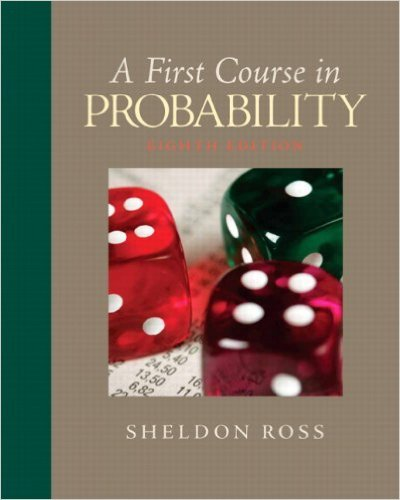×
Get Full Access to First Course In Probability - 8 Edition - Chapter 6 - Problem 6.48
Get Full Access to First Course In Probability - 8 Edition - Chapter 6 - Problem 6.48

×

# If X1,X2,X3,X4,X5 are independent andISBN: 9780136033134 163

## Solution for problem 6.48 Chapter 6

First Course in Probability | 8th Edition

• Textbook Solutions
• 2901 Step-by-step solutions solved by professors and subject experts
• Get 24/7 help from StudySoup virtual teaching assistantsFirst Course in Probability | 8th Edition

4 5 1 427 Reviews
31
4
Problem 6.48

If X1,X2,X3,X4,X5 are independent and identicallydistributed exponential random variableswith the parameter , compute(a) P{min(X1, . . . ,X5) a};(b) P{max(X1, . . . ,X5) a}.

Step-by-Step Solution:
Step 1 of 3

Color Coding: Topics MINITAB Examples STT 351 Notes Normal −1 2 f x = 1 e 2 x ( ) 2Π ­inf < x < inf √ To find probabilities between two points (a,b] we have to integrate this function between a,b. Using MINITAB: 1) Calc 2) Probability distribution 3) Normal (mean = 0, Standard deviation = 1) 4) Select cumulative probability This will give the area under the curve up to the point x For any a value, the area under the curve up to the point a is = P(X ≤ a) # If we want to find the area under the curve between two points we can use the equation….. P( a < X ≤ b ) = P( X ≤ b ) – P( X ≤ a ) Using MINITAB: To find

Step 2 of 3

Step 3 of 3

##### ISBN: 9780136033134

The full step-by-step solution to problem: 6.48 from chapter: 6 was answered by , our top Statistics solution expert on 11/23/17, 05:06AM. Since the solution to 6.48 from 6 chapter was answered, more than 320 students have viewed the full step-by-step answer. This textbook survival guide was created for the textbook: First Course in Probability, edition: 8. This full solution covers the following key subjects: compute, distributed, Exponential, identically, independent. This expansive textbook survival guide covers 10 chapters, and 495 solutions. First Course in Probability was written by and is associated to the ISBN: 9780136033134. The answer to “If X1,X2,X3,X4,X5 are independent and identicallydistributed exponential random variableswith the parameter , compute(a) P{min(X1, . . . ,X5) a};(b) P{max(X1, . . . ,X5) a}.” is broken down into a number of easy to follow steps, and 25 words.

Unlock Textbook Solution